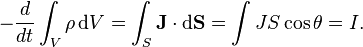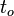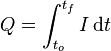Electric charge

ElectromagnetismElectricity ·Magnetism
Electrostatics
Electric charge · Coulomb’s law · Electric flux · Gauss’ law · Electric potential · Electrostatic induction · Electric dipole moment ·

Electric charge is a fundamental, conserved property of some subatomic particles, such as electrons and protons. There are two opposite types of electric charge, known as positive and negative. The charge on an electron is, by convention, -1, and that on a proton is +1. A pair of oppositely charged particles attract each other, and conversely, a pair of pair of particles with the same type of charge repel each other.

An atom is electrically neutral (that is, its net electric charge is zero) because the number of protons within its nucleus is equal to the number of electrons surrounding the nucleus. If an atom gains an extra electron, it acquires a charge of -1; if it loses an electron, it acquires a charge of +1. An electrically charged atom (or group of atoms) is called an ion. Thus, an understanding of electric charge helps clarify the behavior of atoms, molecules, ions, and subatomic particles.

Moreover, electrically charged matter is influenced by, and produces, electromagnetic fields. The interaction between a moving charge and an electromagnetic field is the source of the electromagnetic force (or electromagnetic interaction), which is one of the four fundamental forces.

Overview

Electric charge is a characteristic of some subatomic particles. It is quantized in that, when expressed in units of the so-called elementary charge e (which is the charge on a single electron or a single proton), it takes integer or fractional values. As mentioned above, an electron is said to have a charge of −1, while a proton has the opposite charge of +1. Quarks have a fractional charge of −13 or +23. The antiparticle equivalents of these (positrons, antiprotons, and antiquarks, respectively) have the opposite charge. There are other charged particles. The discrete nature of electric charge was proposed by Michael Faraday in his electrolysis experiments, and then directly demonstrated by Robert Millikan in his oil-drop experiment.

In general, same-sign charged particles repel one another, while different-sign charged particles attract. This is expressed quantitatively in Coulomb's law, which states that the magnitude of the electrostatic repelling force between two particles is proportional to the product of their charges and the inverse square of the distance between them.

The electric charge of a macroscopic object is the sum of the electric charges of its constituent particles. Often, the net electric charge is zero, because it is favorable for the number of electrons in every atom to equal the number of protons (or, more generally, for the number of anions, or negatively charged atoms, in every molecule to equal the number of cations, or positively charged atoms). When the net electric charge is non-zero and motionless, one has the phenomenon known as static electricity. Even when the net charge is zero, it can be distributed non-uniformly (e.g., due to an external electric field, or due to molecular motion), in which case the material is said to be polarized. The charge due to the polarization is known as bound charge, while the excess charge brought from outside is called free charge. The motion of charged particles (e.g., of electrons in metals) in a particular direction is known as electric current.

Units

The SI unit of quantity of electric charge is the coulomb, which is equivalent to about 6.25 × 1018 e (the charge on a single electron or proton). Hence, the charge of an electron is approximately −1.602 x 10−19 C. The coulomb is defined as the quantity of charge that has passed through the cross-section of an electrical conductor carrying one ampere within one second. The symbol Q is often used to denote a quantity of electricity or charge. The quantity of electric charge can be directly measured with an electrometer, or indirectly measured with a ballistic galvanometer.

After finding the quantized character of charge, in 1891 Stoney proposed the unit 'electron' for this fundamental unit of electrical charge. This was before the discovery of the particle by J.J. Thomson in 1897. Today, the name "electron" for the unit of charge is no longer widely used except in the derived unit "electronvolt." This is quite surprising considering the wide use of this unit in the fields of physics and chemistry. The unit is today treated as nameless, referred to as "fundamental unit of charge" or simply as "e."

Formally, a measure of charge should be a multiple of the elementary charge e (charge is quantized), but since it is an average, macroscopic quantity, many orders of magnitude larger than a single elementary charge, it can effectively take on any real value. Furthermore, in some contexts it is meaningful to speak of fractions of a charge; e.g. in the charging of a capacitor.

History

As reported by the Ancient Greek philosopher Thales of Miletus around 600 B.C.E., charge (or electricity) could be accumulated by rubbing fur on various substances, such as amber. The Greeks noted that the charged amber buttons could attract light objects such as hair. They also noted that if they rubbed the amber for long enough, they could even get a spark to jump. This property derives from the triboelectric effect.

In 1600, the English scientist William Gilbert returned to the subject in De Magnete, and coined the New Latin word electricus from the Greek term ηλεκτρον (elektron), meaning "amber." This terminology soon led to the English words "electric" and "electricity." Gilbert's work was followed in 1660 by Otto von Guericke, who invented what was probably the first electrostatic generator.

Other European pioneers were Robert Boyle, Stephen Gray, and C. F. DuFay. In 1675, Boyle stated that electric attraction and repulsion can act across a vacuum. In 1729, Gray classified materials as conductors and insulators. In 1733, DuFay proposed that electricity came in two varieties that canceled each other and expressed this in terms of a two-fluid theory. DuFay said that when glass was rubbed with silk, the glass was charged with vitreous electricity, and when amber was rubbed with fur, the amber was charged with resinous electricity.

In 1839, Michael Faraday showed that the apparent division between static electricity, current electricity, and bioelectricity was incorrect, and all were a consequence of the behavior of a single kind of electricity appearing in opposite polarities. It is arbitrary which polarity one calls positive and which one calls negative. Positive charge can be defined as the charge left on a glass rod after being rubbed with silk.

One of the foremost experts on electricity in the eighteenth century was Benjamin Franklin, who argued in favor of a one-fluid theory of electricity. Franklin imagined electricity as being a type of invisible fluid present in all matter; for example he believed that it was the glass in a Leyden jar that held the accumulated charge. He posited that rubbing insulating surfaces together caused this fluid to change location, and that a flow of this fluid constitutes an electric current. He also posited that when matter contained too little of the fluid it was "negatively" charged, and when it had an excess it was "positively" charged. Arbitrarily (or for a reason that was not recorded), he identified the term "positive" with vitreous electricity and "negative" with resinous electricity. William Watson arrived at the same explanation at about the same time.

Electrification by friction

The following experiment demonstrates electrification by friction.

Take a piece of glass and a piece of resin that do not exhibit any electrical properties. If they are rubbed against each other and the rubbed surfaces are kept in contact with each other, they will still exhibit no electrical properties. If they are separated, however, they will attract each other.

Rub a second piece of glass against a second piece of resin and separate them. If these two pieces are suspended near the first two pieces of glass and resin, it may be observed that:

1. the two pieces of glass repel each other;
2. the two pieces of resin repel each other; and
3. each piece of glass attracts each piece of resin.

These properties of attraction and repulsion are called electrical phenomena, and the bodies exhibiting them are said to be 'electrified' or 'charged with electricity'. Bodies may be electrified in many other ways, as well as by friction.

The electrical properties of the two pieces of glass are similar to each other but opposite to those of the two pieces of resin: the glass attracts what the resin repels and repels what the resin attracts.

Following DuFay's terminology (noted above), if an electrified body behaves as the glass does, (that is, it repels the glass and attracts the resin), the body is said to be 'vitreously' electrified; and if it attracts the glass and repels the resin, it is said to be 'resinously' electrified. All electrified bodies are found to be either vitreously or resinously electrified. By convention, the vitreous electrification is called positive, and the resinous electrification, negative. No force, of either attraction or repulsion, can be observed between an electrified body and a body not electrified.

We now know that there is only one kind of electrical charge, and only one variable is required to keep track of the amount of charge. However, just knowing the charge of a particle is not a complete description of the situation. Matter is composed of several kinds of electrically charged particles, and these particles have many properties, not just charge.

The most common charge carriers are the positively charged proton and the negatively charged electron. The movement of any of these charged particles constitutes an electric current. In many situations, it suffices to speak of the conventional current without regard to whether it is carried by positive charges moving in the direction of the conventional current and/or by negative charges moving in the opposite direction. This macroscopic viewpoint is an approximation that simplifies electromagnetic concepts and calculations.

At the opposite extreme, if one looks at the microscopic situation, one sees there are many ways of carrying an electric current, including: a flow of electrons; a flow of electron "holes" which act like positive particles; and both negative and positive particles (ions or other charged particles) flowing in opposite directions in an electrolytic solution or a plasma).

It should be noted that when electrical current is carried by a metallic wire, the direction of the conventional current is opposite the flow of the actual charge carriers, that is, the electrons.

Properties

Aside from the properties described in articles about electromagnetism, charge is a relativistic invariant. This means that any particle that has charge Q, no matter how fast it goes, always has charge Q. This property has been experimentally verified by showing that the charge of one helium nucleus (two protons and two neutrons bound together in a nucleus) moving at high speeds is the same as that of two deuterium nuclei (one proton and one neutron bound together) moving much more slowly.

Conservation of charge

The total electric charge of an isolated system remains constant regardless of changes within the system itself. This law is inherent to all processes known to physics. The conservation of charge results in the charge-current continuity equation. More generally, the net change in charge density$\rho$ within a volume of integration$V$ is equal to the area integral over the current density$J$ on the surface of the area$S$, which is in turn equal to the net current$I$:$- \frac{d}{dt} \int_V \rho\, \mathrm{d}V = \int_S \mathbf{J} \cdot \mathrm{d}\mathbf{S} = \int J S \cos\theta = I.$

Thus, the conservation of electric charge, as expressed by the continuity equation, gives the result:$I = \frac{dQ}{dt}.$

The charge transferred between time$t_o$ and$t$ is obtained by integrating both sides:$Q = \int_{t_o}^{t_f} I\, \mathrm{d}t$

where I is the net outward current through a closed surface and Q is the electric charge contained within the volume defined by the surface.